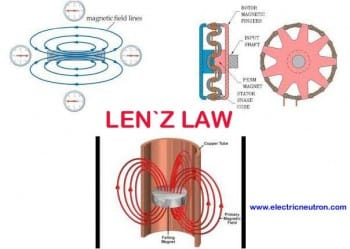# Single Phase AC Motor

### Basic principles of operation

Electromagnetic field generated from the stator does not rotate.It is a repeater between the poles as the poles alternating ac voltage changes polarity.

The induced voltage in the rotor is the result of magnetic induction, and then the magnetic field produced around the rotor.This field is opposite to the stator field (Len `z Law).### The types of single phase AC motor

There are four types of single phase ac motor is used as follows:

1) Split Phase Motor

2) Start resistor Motor

3) Capacitor Motor

I will explain detail in my next post about four ( 4 ) type of single phase AC motor.

How to calculate single phase motor speed?

Speed of an induction motor depends on the motor design itself, the synchronous speed determined by the frequency and amount of AC input power poles in the stator.

the higher the number of poles, the slower synchronous speed.Relationship between poles, frequency and speed of synchronous is as follows:

Ns(rpm) = 120 x f /p

Ns = synchronous speed

f = supply frequency

p = number of poles in the stator

example :

a four ( 4 ) pole motor is built to operate at 60 Hz and synchronous speed is:

Ns = 120 x f /p

Ns = 120 x 60 / 4

Ns = 1800 rpm

### Slip in electric motor

The difference in rotor speed and synchronous speed is known as slip

we can calculate the slip of a motor using the formula:

S = Ns – Nr / Ns x 100%

S = slip

Ns =stator magnetic field speed

Nr = rotor speed (available on the motor name plate)

### How to calculate HORSE POWER for motor?

To calculate the total power output of a motor, we can use the formula:

HP = I x V x Eff / 746

I = Ampere

V = voltage

Eff = efficiency (available on the motor name plate)

Example :

1 unit single phase motor,240 volt,20 ampere and 85% efficiency

HP = I x V x Eff / 746

HP = 20 x 240 x 85% / 746

HP = 5.5

1.vishal patel says

how to mesure single pahse induction motors resistance.
plz help me.

2.divyaprakash sharma says

what will be the stator resistance of 1.5 hp 3phse induction motor or tell me the formula to calculate that ?

3.Berman Strat says

Hi, I’m glad with your great help on How to use the Multimeter.
with a clearer video.

Thanks.
Strat.

4.Chris says

Hi Lemau, very helpful post, thanks for explaining this in such a clear way. Laying out he equations is definitely the best way to figure this out, thanks for that. Keep up the posts!

5.waleed ahmed atiq says

from where 120 is coming ??

This site uses Akismet to reduce spam. Learn how your comment data is processed.